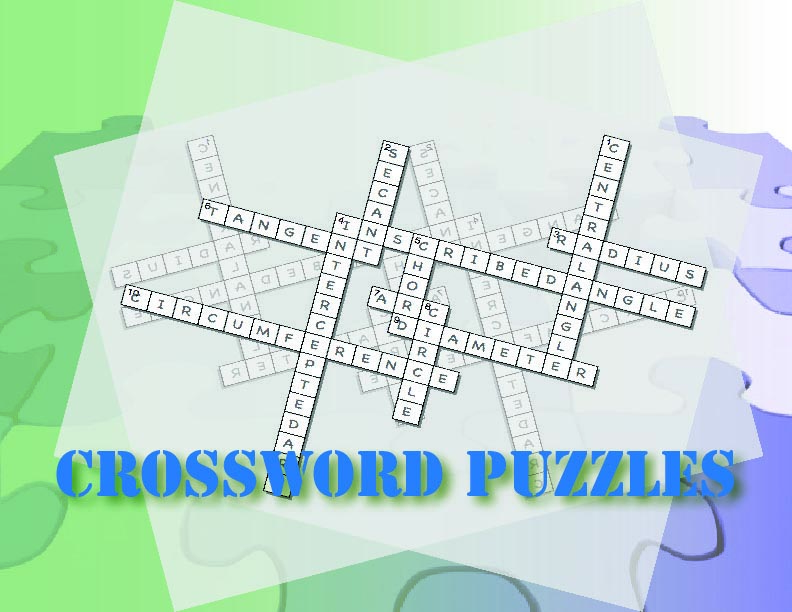# Unit: Equations### Key topics include:

• Equations, including true, false, and conditional equations
• Properties of Equality
• Solving One-Step Equations

### The suggested timeframe for each lesson can be adapted to suit your needs.### Days 1-2: Speaking Math

Introduction of key vocabulary around equations. This is a gamified experience with word puzzles and other games.

Introduce these terms and definitions

• Equation
• Equality
• Solution
• True Equation
• False Equation
• Algebraic Expression
• Equals Sign
• Conditional Equation
• The Unknown

Media4Math Resources:

Goal: Gain an understanding of the key words used throughout this unit.### Days 3-4: Building Blocks

Introduction to the properties of equality:

• Subtraction Property of Equality
• Multiplication Property of Equality
• Division Property of Equality

Media4Math Resources:

Goal: To develop a concrete understanding of equations and the properties of equality. Establishes that math is not just computation, but logical reasoning.### Days 5-6: Putting it Together

Learn the mechanics of solving one-step equations.

Media4Math Resources:

Goal: Develop mastery at solving one-step equations using the properties of equality.### Days 7-8: Show What You Know

A review of key concepts, specifically solving one-step equations.

Media4Math Resources:

Goal: Ability to solve one-step equations involving addition, subtraction, multiplication, and division.